# Rethinking the graph convolutional network GNN is just a filter

Share
/ 2019-06-05 / News

The latest research suggests that the graph neural network only performs low-pass filtering on eigenvectors and does not have nonlinear manifold learning characteristics. The paper proposes a theoretical framework based on graphics signal processing for analyzing graph neural networks.

Graphic neural network has become one of the most important techniques for solving the problem of graph structure data learning.

Recent work on vertex classification (vertex classification) presents a deep and distributed learning model for high performance And scalability.

But recently,A paper entitled "Revisiting Graph Neural Networks: All We Have is Low-Pass Filters" has drawn attention, and it is proposed that the graph neural network is only The eigenvectors are low pass filtered.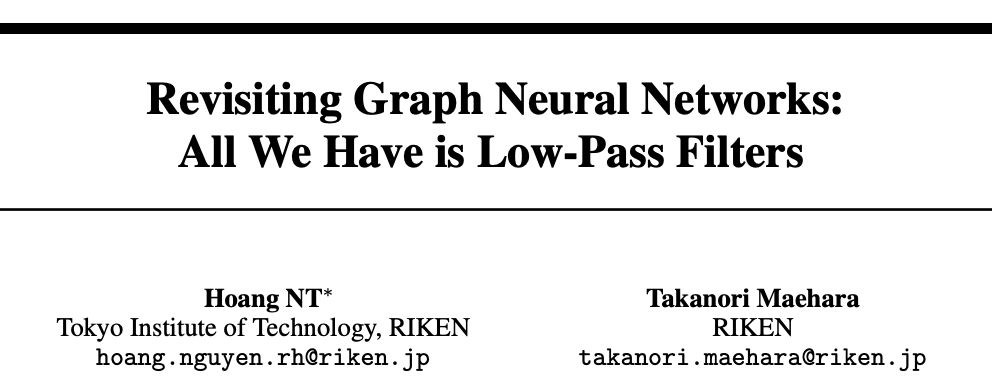Two researchers from Tokyo Institute of Technology and RIKEN found that the eigenvectors of the benchmark dataset already provide a lot of useful information for the classification task. The graph structure only provides a way to de-dry the data.

The paper proposes a theoretical framework based on graphical signal processing for analyzing graph neural networks.

The authors stated that their results indicate that the graph neural network only performs low-pass filtering on eigenvectors and does not have nonlinear manifold learning. characteristic. The paper further studies their adaptive stress on characteristic noise, and puts forward some insights on the design of graph neural network based on GCN.

When should I use a graph neural network?

Graph neural networks (GNN) is a type of neural network that can learn from graph structure data. In recent years, graph neural networks for vertex classification and graphical isomorphism have achieved good results on multiple benchmark datasets.Does not break the most advanced technical performance of innovation. With the success of ChebNet and GCN in vertex classification, many GNN variants have been proposed to address social networking, biology, chemistry, natural language processing, computer vision, and weakly supervised learning.

In the semi-supervised vertex classification problem, we observed that the graph convolutional layer (GCN) parameters only lead to overfitting. Similar observations have been reported in simple architectures (such as SGC) and more complex foot bones (such as DGI).

Based on this phenomenon, Felix Wu et al. proposed simply viewing the graph neural network as feature propagation and proposing a An efficient model with the most advanced performance on the benchmark dataset. Kawamoto et al. conducted a related theoretical review of untrained GCN-like GNNs under the partitioning of graphs.

From these previous studies, a very natural question arises: Why, and when the graph neural network performs well in vertex classification tasks?

In other words, is there a condition for a vertex feature vector that makes the graph neural network model work well even without training?

So, we Can you find a practical counterexample to the failure of a baseline neural network (such as SGC or GCN)?

In this study, we answer the above from the perspective of signal processing. Problem. In form, we consider the semi-supervised learning problem of a graph.

Given a graph G = (V, E), each vertex i ∈V has a characteristic x(i)∈x, and labely(i)∈y, where x is the d-dimensional Euclidean space R d, Y = R is used for regression, Y ={ 1,…,c} is used for classification. The task is to learn the hypothesis of predicting the label y(i) from the feature x(i).

Then we describe the graph neural network solution for this problem and provide the mechanism for the most commonly used benchmark model GCN and its simplified variant SGC. opinion.

Three contributions to this study

Graph signal processing (GSP) will The data at the vertices is treated as a signal, and signal processing techniques are applied to understand the characteristics of the signal. GSP inspired the development of graph structure data learning algorithms by combining signals (feature vectors) and graph structures (conversions of adjacency matrices or adjacency matrices). In standard signal processing problems, it is generally assumed that the observations contain some noise and the underlying "true signal" has low frequencies. Here, we make similar assumptions about our problems.

Assumption 1: Input features include low frequency real features and noise. Real features provide sufficient information for machine learning tasks.

The first contribution of this study It is a hypothesis 1 (Section 3) that validates common data sets. Figure 1 shows the performance of Layer 2 Perceptrons (MLPs) trained for the characteristics of different frequency components. In all benchmark data sets, we see Only a few frequency components contribute to learning. Adding more frequency components to the eigenvector will only result in performance degradation. Conversely, when we add Gaussian noise N (0, σ2 ) to the feature, the classification accuracy becomes more Worse.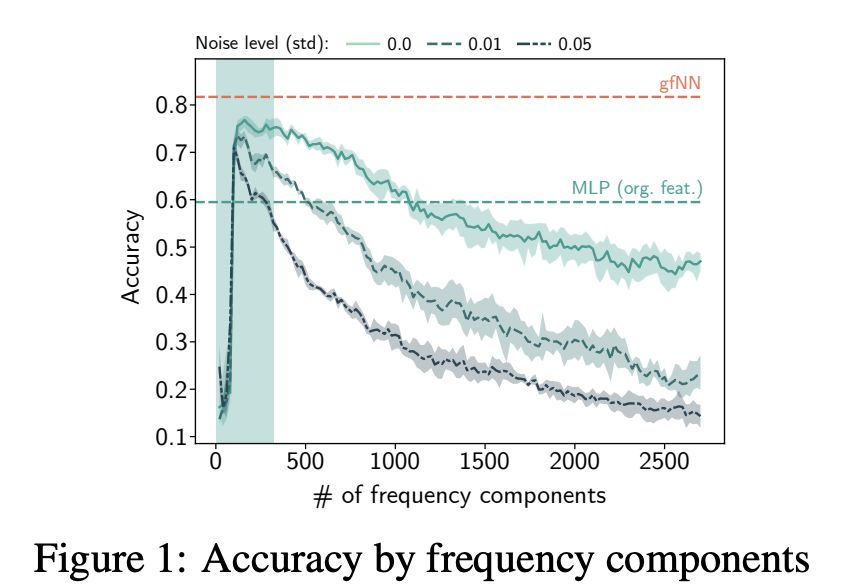Figure 1: Accuracy of frequency components

A lot of recent GNNs are built Based on the signal processing of the graph, the most common practice is to normalize the characteristics of the adjacency matrix I − L ̃ and matrix X by (enhancement). In the literature of signal processing, this operation filters the signals on the graph. ), without explicitly decomposing the normalized Laplacian matrix. Here, we refer to this enhanced normalized adjacency matrix and its variants as interchangeable graph filters ( Graph filters) and propagation matrices.

The second contribution of this study suggests that multiplying the graph signal by the propagation matrix corresponds to low-pass filtering. (Section 4, especially Theorem 3), in addition, we also prove that the matrix product between the observed signal and the low-pass filter is an analytical solution to the real signal optimization problem.Compared with the recent diagram neural network design principle, our results show that the graph convolution layer is only low-pass filtering. Therefore, there is no need to learn the parameters of the graph convolutional layer.

Based on theoretical understanding, we propose a new benchmark framework called gfNN ((graph filter neural network), An empirical analysis of vertex classification problems is performed.

gfNN consists of two steps:

Filter characteristics are achieved by multiplication with the graph filter matrix;

learn vertex labels through machine learning models.

We demonstrate the effectiveness of the framework using a simple implementation model in Figure 2.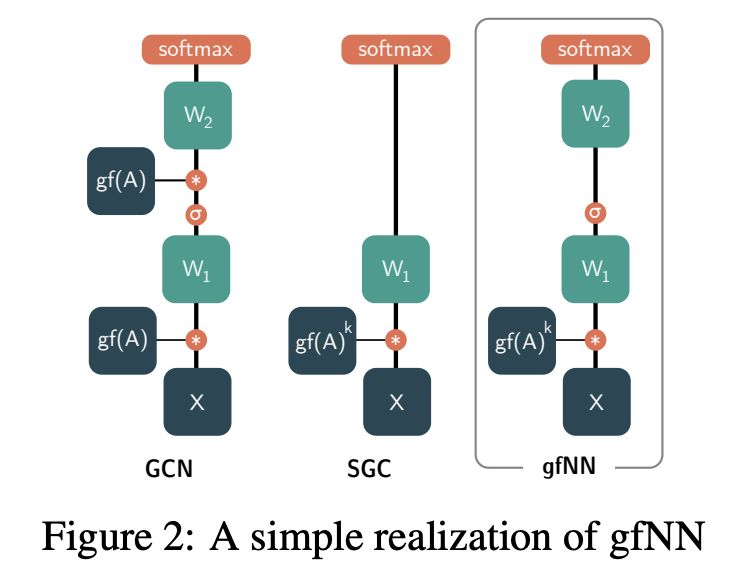Figure 2: A simple implementation of gfNN

The third of this study The contribution is the following theorem:

Theorem 2: Under Hypothesis 1, the results of SGC, GCN, and gfNN are similar to those of the corresponding neural network using real features.

Theorem 7 shows that under hypothesis 1, gfNN and GCN have similar high performance.Since gfNN does not require multiplication of adjacency matrices during the learning phase, it is much faster than GCN. In addition, gfNN is more tolerant of noise.

Finally, we compare gfNN with the SGC model. Although the SGC is fast and accurate on the benchmark dataset, our analysis shows that when the feature input is nonlinearly separable, the SGC will fail because the graph convolution portion does not contribute to nonlinear manifold learning. To prove this point empirically, we created a manual data set.

Experiment and Results

In order to verify the ideas presented earlier, we designed two experiments. . In Experiment E1, we added different levels of white noise to the feature vectors of the real dataset and compared the classification accuracy of different baseline models.

In Experiment E2,We studied an artificial data set with complex feature spaces to prove that simple models such as SGC would fail when sorting.

Table 1 gives an overview of each data set.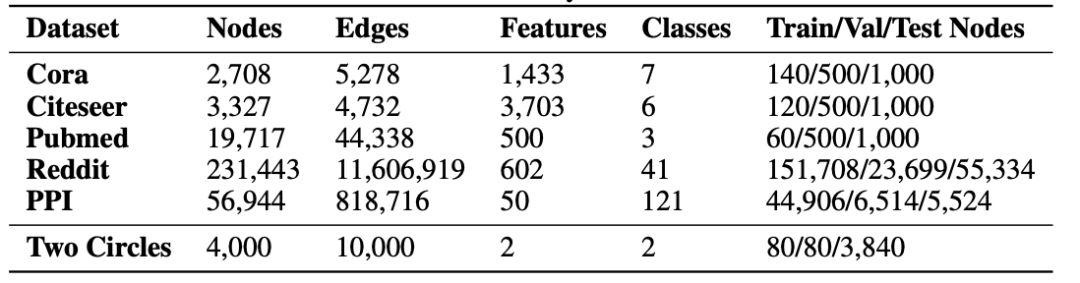Table 1: Actual benchmark datasets for vertex categorization and synthesis datasets

neural networkFigure 4: Cora Benchmark accuracy on the (left), Citeseer (middle), and Pubmed (right) data sets. The noise level is measured by adding the standard deviation of white noise to the eigenvalues.

Denoising effect of image filter

For each data set, we introduce a white noise N(0, 2) as a feature In the range of vector? (0.01, 0.05). According to the meaning of Theorem 8 and Theorem 7, due to the first-order denoising property of GCN, its tolerance to characteristic noise is low.

As the noise level increases,As we can see in Figure 4, GCN, Logistic Regression (LR) and MLP are easier to overfit the noise. On the other hand, gfNN and SGC have similar tolerance to noise.

Expression of graph filters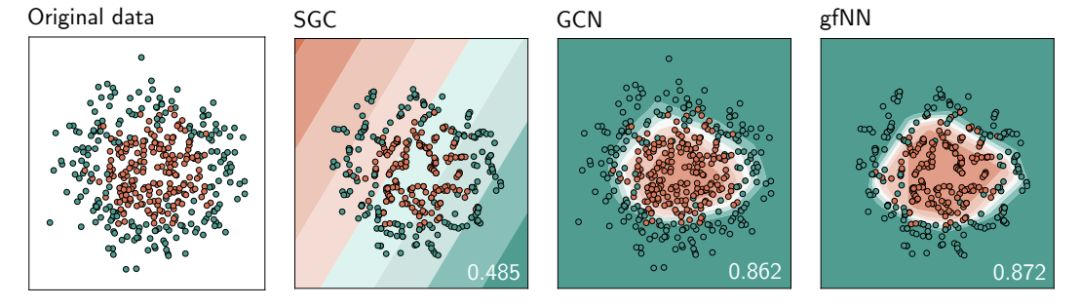Figure 5: Based on two circles The decision boundary of the 500 data samples generated by the patternTable 2: Random train/val/test The average test accuracy of the segment (5 times)

summary

Several work involves the limitations of the GCN architecture. Kawamoto et al. used the mean field method to perform a statistical physical analysis of a simple GCN model. They concluded that backpropagation does not improve GCN-based The accuracy of the GNN model does not improve its detectability. Li et al. conducted an empirical analysis of the multi-layer GCN model with limited label data settings, indicating that if the label data is too small or there are too many overlays, GCN Performance will drop.While these results provide insightful insights into GCN, they do not adequately answer this question: When should we use GNN?

Our The results show that if Hypothesis 1 is established, we should use the GNN method to solve the given problem. From our perspective, GNNs derived from GCN simply perform noise filtering and learn from denoised data.

Based on our analysis, we propose two scenarios that GCN and SGC may not be able to perform: noise characteristics and nonlinear feature space. Then we come up with a simple way to work well in both cases.

In recent years, GCN-based neural networks have been widely used in point cloud analysis and weak supervised learning. With the complexity of the input feature space, we propose to revisit the current GCN-based GNNs design. In computer vision, the GCN layer is not a convolutional layer.We need to think of it as a denoising mechanism. Therefore, simply superimposing the GCN layer will only introduce over-fitting and complexity to the neural network design.

Relevant articles recommend

Top suppliers

Product:Photo Coupler,Optocoupler,IC,TOS,ON,AVAGO,SHARP,RENESAS,CT,EL,LTV,TI,NXP,ST
1YRS
Product:Infrared Receiver Module,Infrared Emitting Diode,Silicon PIN Photodiode,Silicon Phototransistor,LED,Silicon Photocell,Hall Effect IC,Photoelectric switch,Photoelectric encoder,IC.
1YRS
Product:KSD301 KSD9700 thermostat thermal protector fuse sensor
1YRS
Product:IC,PCB,module,capacitor,resistor,diodes,LED,LCD
1YRS
Product:Electronics components，ICs，MCU，RS-485/RS-422 Transceiver
9YRS
Subscribe News

Enter your email address:

Hot News

Contact Us

+86-755-83536845

One to One Customer Service

17190417227